# Sisters

Sisters Zuzka Lenka and Vierka has save for a new bike. Zuzka save € 90, Lenka save € 90 and Vierka save € 82. Missing 30% gave them their mother. What is the price of the bike?

Correct result:

x =  374.29 Eur

#### Solution: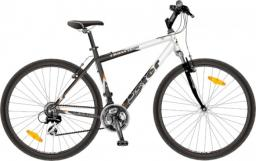We would be pleased if you find an error in the word problem, spelling mistakes, or inaccuracies and send it to us. Thank you!Tips to related online calculators
Do you have a linear equation or system of equations and looking for its solution? Or do you have quadratic equation?

## Next similar math problems:

• SummerjobThe temporary workers planted new trees. Of the total number of 500 seedlings, they managed to plant 426. How many percents did they meet the daily planting limit?
• The Chemistry testThe Chemistry test contained 8 questions, each with 3 points. Peter scored 21 points. How many percent did Peter write a test?
• Percentages52 is what percent of 93?
• Art schoolEvery fifth pupil 9A goes to art school. How many percent of pupils in class 9A go to art school?
• PersonsPersons surveyed:100 with result: Volleyball=15% Baseball=9% Sepak Takraw=8% Pingpong=8% Basketball=60% Find the average how many like Basketball and Volleyball. Please show your solution.
• Reward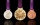Janko and Peter divided the reward from the brigade so that Peter got 5/8 of the reward. What percentage of Janko's reward got?
• GirlsThe children's competition was attended by 63 girls, which is 30% of all children's participants. How many children attended this competition?
• Reducing numberReducing the an unknown number by 28.5% we get number 243.1. Determine unknown number.
• PupilsThere are 350 girls in the school, and the other 30% of the total number of pupils are boys. How many pupils does the school have?
• Jane classWhen asked how many students are in class, Jane said, if we increase the number of students in our class by hundred % and then add half the number of students, we get 100. How many students are in Jane's class?
• Original planGardeners planted 16% more currant bushes than originally planned. In total, they planted x = 435 shrubs. What was the original plan?
• Unknown number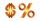15% of the unknown number is 18 less than 21% of the same number. What is the unknown number?
• Perctentages35% of what number is 35?
• Cinema ticketsCinema sold 180 tickets this Thursday, which is 20%. Monday 14%, Tuesday 6%, Wednesday 9%, Friday 24%, Saturday 12%, and Sunday 15%. How many tickets were sold per week?
• StudentsStudents solve word problems. They received 3 points for each correctly solved task. Four points were deducted for a task that was not solved or incorrectly solved. Tomas solved a total of 15 tasks and scored 24 points. How many tasks did he solve correct
• Ice skates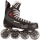Ice skates were raised twice, the first time by 25%, the second time by 10%. After the second price, their cost was 82.5 euros. What was the original price of skates?
• Copy typist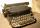Typist for 12 hours rewritten 15% of the manuscript. After how many hours he will done 35% of the manuscript?